The LIFEREG Procedure
 OUTPUT Statement

OUTPUT <OUT=SAS-data-set> <keyword=name> ...<keyword=name> ;

The OUTPUT statement creates a new SAS data set containing statistics calculated after fitting the model. At least one specification of the form keyword=name is required.

All variables in the original data set are included in the new data set, along with the variables created as options for the OUTPUT statement. These new variables contain fitted values and estimated quantiles. If you want to create a permanent SAS data set, you must specify a two-level name (see SAS Language Reference: Concepts for more information about permanent SAS data sets). Each OUTPUT statement applies to the preceding MODEL statement. See Example 48.1 for illustrations of the OUTPUT statement.

The following specifications can appear in the OUTPUT statement:

OUT=SAS-data-set

specifies the new data set. By default, the procedure uses the DATAconvention to name the new data set.

keyword=name

specifies the statistics to include in the output data set and gives names to the new variables. Specify a keyword for each desired statistic (see the following list of keywords), an equal sign, and the variable to contain the statistic.

The keywords allowed and the statistics they represent are as follows:

CENSORED

specifies an indicator variable to signal censoring. The variable takes on the value 1 if the observation is censored; otherwise, it is 0.

CDF

specifies a variable to contain the estimates of the cumulative distribution function evaluated at the observed response. See the section Predicted Values for more information.

CONTROL

specifies a variable in the input data set to control the estimation of quantiles. See Example 48.1 for an illustration. If the specified variable has the value 1, estimates for all the values listed in the QUANTILE= list are computed for that observation in the input data set; otherwise, no estimates are computed. If no CONTROL= variable is specified, all quantiles are estimated for all observations. If the response variable in the MODEL statement is binomial, then this option has no effect.

CRESIDUAL | CRES

specifies a variable to contain the Cox-Snell residuals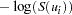where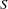is the standard survival function and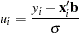If the response variable in the corresponding model statement is binomial, then the residuals are not computed, and this variable contains missing values.

SRESIDUAL | SRES

specifies a variable to contain the standardized residuals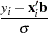If the response variable in the corresponding model statement is binomial, then the residuals are not computed, and this variable contains missing values.

PREDICTED | P

specifies a variable to contain the quantile estimates. If the response variable in the corresponding model statement is binomial, then this variable contains the estimated probabilities,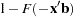.

QUANTILES | QUANTILE | Q

gives a list of values for which quantiles are calculated. The values must be between 0 and 1, noninclusive. For each value, a corresponding quantile is estimated. This option is not used if the response variable in the corresponding MODEL statement is binomial. The QUANTILES option can be specified as follows.

 Type of List Specification list separated by blanks .2 .4 .6 .8 list separated by commas .2,.4,.6,.8 x to y .2 to .8 x to y by z .2 to .8 by .1 combination of methods .1,.2 to .8 by .2

By default, QUANTILES=0.5. When the response is not binomial, a numeric variable, _PROB_, is added to the OUTPUT data set whenever the QUANTILES= option is specified. The variable _PROB_ gives the probability value for the quantile estimates. These are the values taken from the QUANTILES= list and are given as values between 0 and 1, not as values between 0 and 100.

STD_ERR | STD

specifies a variable to contain the estimates of the standard errors of the estimated quantiles or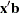. If the response used in the MODEL statement is a binomial response, then these are the standard errors of. Otherwise, they are the standard errors of the quantile estimates. These estimates can be used to compute confidence intervals for the quantiles. However, if the model is fit to the log of the event time, better confidence intervals can usually be computed by transforming the confidence intervals for the log response. See Example 48.1 for such a transformation.

XBETA

specifies a variable to contain the computed value of, whereis the covariate vector and b is the vector of parameter estimates.Previous Page | Next Page | Top of Page